Search by Topic

Resources tagged with Visualising similar to Paving Paths:

Filter by: Content type:
Age range:
Challenge level:

There are 176 results

Broad Topics > Using, Applying and Reasoning about Mathematics > VisualisingPaving Paths

Age 11 to 14 Challenge Level:

How many different ways can I lay 10 paving slabs, each 2 foot by 1 foot, to make a path 2 foot wide and 10 foot long from my back door into my garden, without cutting any of the paving slabs?Rati-o

Age 11 to 14 Challenge Level:

Points P, Q, R and S each divide the sides AB, BC, CD and DA respectively in the ratio of 2 : 1. Join the points. What is the area of the parallelogram PQRS in relation to the original rectangle?Tetra Square

Age 11 to 14 Challenge Level:

ABCD is a regular tetrahedron and the points P, Q, R and S are the midpoints of the edges AB, BD, CD and CA. Prove that PQRS is a square.Coordinate Patterns

Age 11 to 14 Challenge Level:

Charlie and Alison have been drawing patterns on coordinate grids. Can you picture where the patterns lead?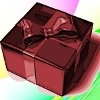Christmas Boxes

Age 11 to 14 Challenge Level:

Find all the ways to cut out a 'net' of six squares that can be folded into a cube.Khun Phaen Escapes to Freedom

Age 11 to 14 Challenge Level:

Slide the pieces to move Khun Phaen past all the guards into the position on the right from which he can escape to freedom.Coloured Edges

Age 11 to 14 Challenge Level:

The whole set of tiles is used to make a square. This has a green and blue border. There are no green or blue tiles anywhere in the square except on this border. How many tiles are there in the set?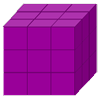Cubist Cuts

Age 11 to 14 Challenge Level:

A 3x3x3 cube may be reduced to unit cubes in six saw cuts. If after every cut you can rearrange the pieces before cutting straight through, can you do it in fewer?Triangle Inequality

Age 11 to 14 Challenge Level:

ABC is an equilateral triangle and P is a point in the interior of the triangle. We know that AP = 3cm and BP = 4cm. Prove that CP must be less than 10 cm.An Unusual Shape

Age 11 to 14 Challenge Level:

Can you maximise the area available to a grazing goat?Age 11 to 14 Challenge Level:

Four rods, two of length a and two of length b, are linked to form a kite. The linkage is moveable so that the angles change. What is the maximum area of the kite?Framed

Age 11 to 14 Challenge Level:

Seven small rectangular pictures have one inch wide frames. The frames are removed and the pictures are fitted together like a jigsaw to make a rectangle of length 12 inches. Find the dimensions of. . . .Concrete Wheel

Age 11 to 14 Challenge Level:

A huge wheel is rolling past your window. What do you see?Take Ten

Age 11 to 14 Challenge Level:

Is it possible to remove ten unit cubes from a 3 by 3 by 3 cube so that the surface area of the remaining solid is the same as the surface area of the original?Counting Triangles

Age 11 to 14 Challenge Level:

Triangles are formed by joining the vertices of a skeletal cube. How many different types of triangle are there? How many triangles altogether?Hidden Rectangles

Age 11 to 14 Challenge Level:

Rectangles are considered different if they vary in size or have different locations. How many different rectangles can be drawn on a chessboard?The Old Goats

Age 11 to 14 Challenge Level:

A rectangular field has two posts with a ring on top of each post. There are two quarrelsome goats and plenty of ropes which you can tie to their collars. How can you secure them so they can't. . . .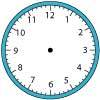Right Time

Age 11 to 14 Challenge Level:

At the time of writing the hour and minute hands of my clock are at right angles. How long will it be before they are at right angles again?How Many Dice?

Age 11 to 14 Challenge Level:

A standard die has the numbers 1, 2 and 3 are opposite 6, 5 and 4 respectively so that opposite faces add to 7? If you make standard dice by writing 1, 2, 3, 4, 5, 6 on blank cubes you will find. . . .Pattern Power

Age 5 to 14

Mathematics is the study of patterns. Studying pattern is an opportunity to observe, hypothesise, experiment, discover and create.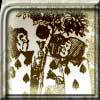Painting Cubes

Age 11 to 14 Challenge Level:

Imagine you have six different colours of paint. You paint a cube using a different colour for each of the six faces. How many different cubes can be painted using the same set of six colours?All in the Mind

Age 11 to 14 Challenge Level:

Imagine you are suspending a cube from one vertex and allowing it to hang freely. What shape does the surface of the water make around the cube?Convex Polygons

Age 11 to 14 Challenge Level:

Show that among the interior angles of a convex polygon there cannot be more than three acute angles.Sea Defences

Age 7 to 14 Challenge Level:

These are pictures of the sea defences at New Brighton. Can you work out what a basic shape might be in both images of the sea wall and work out a way they might fit together?Bands and Bridges: Bringing Topology Back

Age 7 to 14

Lyndon Baker describes how the Mobius strip and Euler's law can introduce pupils to the idea of topology.Zooming in on the Squares

Age 7 to 14

Start with a large square, join the midpoints of its sides, you'll see four right angled triangles. Remove these triangles, a second square is left. Repeat the operation. What happens?Getting an Angle

Age 11 to 14 Challenge Level:

How can you make an angle of 60 degrees by folding a sheet of paper twice?Polygon Rings

Age 11 to 14 Challenge Level:

Join pentagons together edge to edge. Will they form a ring?Dissect

Age 11 to 14 Challenge Level:

What is the minimum number of squares a 13 by 13 square can be dissected into?Eight Hidden Squares

Age 7 to 14 Challenge Level:

On the graph there are 28 marked points. These points all mark the vertices (corners) of eight hidden squares. Can you find the eight hidden squares?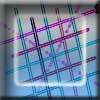Tic Tac Toe

Age 11 to 14 Challenge Level:

In the game of Noughts and Crosses there are 8 distinct winning lines. How many distinct winning lines are there in a game played on a 3 by 3 by 3 board, with 27 cells?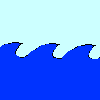Crossing the Atlantic

Age 11 to 14 Challenge Level:

Every day at noon a boat leaves Le Havre for New York while another boat leaves New York for Le Havre. The ocean crossing takes seven days. How many boats will each boat cross during their journey?There and Back Again

Age 11 to 14 Challenge Level:

Bilbo goes on an adventure, before arriving back home. Using the information given about his journey, can you work out where Bilbo lives?Age 11 to 14 Challenge Level:

Can you mark 4 points on a flat surface so that there are only two different distances between them?Squares in Rectangles

Age 11 to 14 Challenge Level:

A 2 by 3 rectangle contains 8 squares and a 3 by 4 rectangle contains 20 squares. What size rectangle(s) contain(s) exactly 100 squares? Can you find them all?Chess

Age 11 to 14 Challenge Level:

What would be the smallest number of moves needed to move a Knight from a chess set from one corner to the opposite corner of a 99 by 99 square board?Troublesome Dice

Age 11 to 14 Challenge Level:

When dice land edge-up, we usually roll again. But what if we didn't...?Auditorium Steps

Age 7 to 14 Challenge Level:

What is the shape of wrapping paper that you would need to completely wrap this model?3D Stacks

Age 7 to 14 Challenge Level:

Can you find a way of representing these arrangements of balls?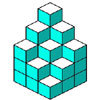Soma - So Good

Age 11 to 14 Challenge Level:

Can you mentally fit the 7 SOMA pieces together to make a cube? Can you do it in more than one way?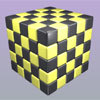Drilling Many Cubes

Age 7 to 14 Challenge Level:

A useful visualising exercise which offers opportunities for discussion and generalising, and which could be used for thinking about the formulae needed for generating the results on a spreadsheet.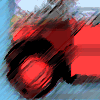Buses

Age 11 to 14 Challenge Level:

A bus route has a total duration of 40 minutes. Every 10 minutes, two buses set out, one from each end. How many buses will one bus meet on its way from one end to the other end?Seven Squares

Age 11 to 14 Challenge Level:

Watch these videos to see how Phoebe, Alice and Luke chose to draw 7 squares. How would they draw 100?Screwed-up

Age 11 to 14 Challenge Level:

A cylindrical helix is just a spiral on a cylinder, like an ordinary spring or the thread on a bolt. If I turn a left-handed helix over (top to bottom) does it become a right handed helix?Cogs

Age 11 to 14 Challenge Level:

A and B are two interlocking cogwheels having p teeth and q teeth respectively. One tooth on B is painted red. Find the values of p and q for which the red tooth on B contacts every gap on the. . . .Christmas Chocolates

Age 11 to 14 Challenge Level:

How could Penny, Tom and Matthew work out how many chocolates there are in different sized boxes?Route to Infinity

Age 11 to 14 Challenge Level:

Can you describe this route to infinity? Where will the arrows take you next?Diminishing Returns

Age 11 to 14 Challenge Level:

How much of the square is coloured blue? How will the pattern continue?Cutting a Cube

Age 11 to 14 Challenge Level:

A half-cube is cut into two pieces by a plane through the long diagonal and at right angles to it. Can you draw a net of these pieces? Are they identical?Cubes Within Cubes

Age 7 to 14 Challenge Level:

We start with one yellow cube and build around it to make a 3x3x3 cube with red cubes. Then we build around that red cube with blue cubes and so on. How many cubes of each colour have we used?